## Mathematics P2 Questions and Answers - Momaliche 4 cycle Post Mock Exams 2021/2022

Instructions to candidates

1. Write your name and index number in the spaces provide above
2. Sign and write the date of examination in the spaces provided above
3. This paper consists TWO section:Section I and Section II.
4. Answer all the Questions in Section I and Five questions from Section II.
5. All answers and workings must be written on this paper.

For Examiners use only
Section I

 1 2 3 4 5 6 7 8 9 10 11 12 13 14 15 16 Total

Section II

 17 18 19 20 21 22 23 24 Total

## QUESTIONS

SECTION 1 : 50 MKS

1. Use logarithm tables only, giving your answer corrected to three significant figures ; to evaluate (4mks)2. Make K the subject of the formula. (3mks)3. Solve for θ in radians given. (3mks)
2 Cos 2θ + 1 = 0 for 0 ≤ θ≤ 2 πC
4. Atieno was asked to round off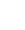to 3 decimal places but she truncated it instead to 3 decimal places. Calculate.
Her percentage error resulting from the misunderstanding. (3mks)
5. Ketepa tea worth Ksh 40 per kg is mixed with Sasini tea worth Ksh 60 per Kg in the ratio 3:1 . In what ratio should this mixture be mixed with Kericho tea worth Ksh 50 per kg to produce a mixture worth Kshs 47 per kg. (3mks)
6. Calculate the length of a chord which is 3cm from the centre of the circle with radius 5cm. . (3mks)
7. The position vectors of point A and B are a= -2i+j-8k and b= -3i+2j-2k respectively . Find the magnitude of AB. (3mks)
8. Without the use of a calculator or mathematical tables, simplify. (3mks)
Sin 330º + Cos 120º
Tan 60º+ Cos 240º
9. The cash price of a music system is Ksh 30000. It can be bought under hire purchase terms by paying a deposit of ksh 10,000 and twelve monthly instalment of Ksh 3200 per month. Determine the percentage rate of interest per month. (3mks)
10. Given that the coefficient of x3 in the expansion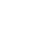4 is 1
1. Find the value of a (2mks)
2. Hence write down the first four terms of4 (2mks)
11. The image of a scalene triangle under the transformation given by the matrixis a straight line. Find the possible value of x (3mks)
12. Use the mid ordinate rule to estimate the area bounded by the curve y+x2 = 4 and the line y = 0 using four strips . (3mks)
13. State the centre and radius of the circle given by the following equation.
(x-4) 2 + (y+2)2 - 4 = 0 (2mks)
14.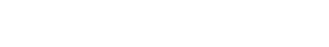Use the line AB above to construct rectangle ABCD with BC = 5cm . A region R moves inside the rectangle under the following conditions
1. It is always nearer to AB than AD
2. and always at least 3cm from point B
Locate R by shading the region. (3mks)
15. The probability that John misses lunch at school is ¾ . If he misses lunch , the probability that he dozes off during the afternoon lessons is 4/7 . Otherwise his probability of dozing off in the afternoon is ½ .
1. Illustrate the probabilities using a tree diagram. (1mk)
2. Calculate the probability that John does not doze off during the afternoon lessons. (2mks)
16. The first 4 terms of a G.P are 4,8,16,32
1. Find the common ratio (1mk)
2. Calculate the sum of the first 8 terms of the G.P (2mks)

SECTION II

1.
1. Complete the table below for the function y = 2 Cos 3x (2mks)
 x 0 100 200 300 400 500 600 700 800 900 1000 1100 1200 Cos 3x 1 - 0.5 -0.5 -1 -1.866 0.5 1 y 2 1 -100 -2 -1.73 1 2
2.
1. Using the values in the completed table, draw the graph of y=2 Cos 3x for 0º≤ x ≤ 120º on the grid provided.2. Using the graph, solve the equation. 2 Cos 3x=0 (3mks)
3. State the amplitude and period of the graph of y= 2 Cos 3x. (2mks)
2. Muma is standing 10 Km from a point P which is due North from her. She walks on a straight on a bearing of 0300 . By calculation, find
1. How far will she have walked when she is equidistant from her starting point and P. (2mks)
2. What is the bearing of P from this equidistant point. (2mk)
3. How far will Muma have walked when she is at the shortest distance from the point p. (2mks)
4. How far will the point P be from Muma when she walked 20 Km on the same straight path. (4mks)
3. The height of a number of orange trees in an orchard were measured to the nearest (cm) and recorded in the table below.
 131 - 140141 – 150151 – 160161 – 170171 – 180181 – 190191 - 200 13233650352815
Using an assumed mean of 165.5, calculate
1. The mean height (3mks)
2. The standard deviation of the distribution (4mks)
3. The quartile deviation (3mks)
4. The points A (5,-1) B (1,-2) and C (x ,y) of a triangle are mapped onto A' (1,5) B'(2,1) and C'(4,2) by a matrix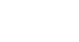Find
1. Matrix N of the transformation. (4mks)
2. Co-ordinates of C (2mks)
3. A" B" C” are the image of A' B'C' under a transformation represented by matrix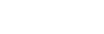Write down the co-ordinates of A" B" C" (2mks)
4. A transformation N followed by M can be represented by a single transformation K. Determine K (2mks)
5. A ship left port A (11º N, 32º W) and sailed due west to another port B. The journey took 160 hours at an average speed of 28 knots. Given that radius of the earth is 6370km and π = 22⁄7
1. Calculate the distance between A and B
1. In nautical miles (2mks)
2. In km (2mks)
2. Calculate the average speed of the ship in km/h correct to 2d.p (2mks)
3. Calculate to the nearest whole number the longitude of port B and hence state its position (4mks)
6.The right pyramid above (not drawn to scale) has AB = 12 cm and BC = 16 cm. O is the centre of the base with OV = 15 cm.
1. The length of the slant edge (2mks)
2. The angle between the lines VA and VC (2mks)
3. The angle between the plane ABV and the base ABCD (3mks)
4. The pyramid is chopped at point T to form a frustum such T divides OV in the ration 2:1. Find the volume of the frustum. (3mks)
7. The figure below shows the sketch of the curve y = x2 - 2x + 4and a straight line PQ which cuts the x-axis and the y-axis at the points (10, 0) (0, 10) respectively.
The line also intersects the curve at P and QFind.
1.
1. The equation of the straight line in the form y = m x + c (2mks)
2. The co-ordinates of P and Q (4mks)
2. Use integration to find the area of the shaded part. (4mks)
8. Owiti makes two types of dresses, A and B. He takes 3 hours to make one pair of type A and 4 hours to make one pair of type B. He works for a maximum of 120 hours to make x pairs of type A and Y pairs of type B. It costs him shs 400 to make a pair of type A and shs. 150 to make a pair of type B. His total cost does not exceed shs. 9000. He must make at least 8 pairs of type A and more than 12 pairs of type B.
1. Write down four inequalities representing the above information. (4mks)
2. On the grid provided, draw the inequalities and shade the unwanted region. (3mks)3. Owiti makes a profit of shs. 40 on each pair of type A and shs. 70 on pair of type B dresses. Use the graph in part (b) above to above determine the maximum possible profit he makes. (3mks)

## MARKING SCHEME

SECTION 1 : 50 MKS

1. Use logarithm tables only, giving your answer corrected to three significant figures ; to evaluate (4mks)No Log 0.52492 83.583√0.3563101 × 3.247=32.5 1.7200        ×21.44001.9221  + 1.36211.5518 = 3 + 2.5518    3         3      31.85051.5115
2. Make K the subject of the formula. (3mks)3. Solve for θ in radians given. (3mks)
2 Cos 2θ + 1 = 0 for 0 ≤ θ≤ 2 πC
2 cos 2θ + 1 = 0
cos 2 θ = -0.5
2θ = 120º,240º,480º,600º
θ= 60º, 120º, 240º
π , 2π, 4π
3   3     3
4. Atieno was asked to round offto 3 decimal places but she truncated it instead to 3 decimal places. Calculate.
Her percentage error resulting from the misunderstanding. (3mks)= 4.4666667
actual = 4.4667
truncation = 4.467
error = 4.467 - 4.66 = 0.001
%error = 0.001 × 100
4.4667
= 0.0223863%
5. Ketepa tea worth Ksh 40 per kg is mixed with Sasini tea worth Ksh 60 per Kg in the ratio 3:1 . In what ratio should this mixture be mixed with Kericho tea worth Ksh 50 per kg to produce a mixture worth Kshs 47 per kg. (3mks)
40 × 3 + 60 × 1 = 120 + 60 = 45/= per kg
4                  4=3:2
6. Calculate the length of a chord which is 3cm from the centre of the circle with radius 5cm. . (3mks)AB = √52 - 32
= 4
length =  4 × 2
= 8 cm
7. The position vectors of point A and B are a= -2i+j-8k and b= -3i+2j-2k respectively . Find the magnitude of AB. (3mks)
AB = AO + OB
=(2i - j + 8k) + (-3i + 2j - 2k)
= -i + j + 6k
(AB) = √(-1)2 + (1)2 + (6)2
= √38
= 6.164
8. Without the use of a calculator or mathematical tables, simplify. (3mks)
Sin 330º + Cos 120º
Tan 60º+ Cos 240ºtan 60º = √3
Cos 240º = -½9. The cash price of a music system is Ksh 30000. It can be bought under hire purchase terms by paying a deposit of ksh 10,000 and twelve monthly instalment of Ksh 3200 per month. Determine the percentage rate of interest per month. (3mks)
total monthly installments = 3200 × 12
= 38400
principal = 30000 - 10000
= 20000
38400 = 20000 (1 + r/100)12
log 1.92 = 12 log (1 + r/100)
1.059 = 1 + r/100
r = 5.9%
10. Given that the coefficient of x3 in the expansion4 is 1
1. Find the value of a (2mks)
a4 + 2a3x + 3/2a2x2 + ax3/2 + x4/16
a/2 = 1
a = 2
2. Hence write down the first four terms of4 (2mks)
24 + 24x + 3/2x4x2 + x3
16 + 16x + 6x2 + x3
11. The image of a scalene triangle under the transformation given by the matrixis a straight line. Find the possible value of x (3mks)
det = 0
x(x + 1) - 2 = 0
x2 + x - 2 = 0
(x + 1) (x - 2) = 0
x = 1 or +2
12. Use the mid ordinate rule to estimate the area bounded by the curve y+x2 = 4 and the line y = 0 using four strips . (3mks)
 x -1.5 -0.5 0.5 1.5 y 1.75 3.75 3.75 1.75
area = 1(1.75 + 3.75 + 3.75 + 1.75)
= 11sq. units
13. State the centre and radius of the circle given by the following equation.
(x-4) 2 + (y+2)2 - 4 = 0 (2mks)
(x - 4)2 + (y - 2)2 - 4 = 0
center (4,-2)
radius = √42 + (-2)2 + 4
= √24 units
14.Use the line AB above to construct rectangle ABCD with BC = 5cm . A region R moves inside the rectangle under the following conditions
1. It is always nearer to AB than AD2. and always at least 3cm from point B
Locate R by shading the region. (3mks)
15. The probability that John misses lunch at school is ¾ . If he misses lunch , the probability that he dozes off during the afternoon lessons is 4/7 . Otherwise his probability of dozing off in the afternoon is ½ .
1. Illustrate the probabilities using a tree diagram. (1mk)2. Calculate the probability that John does not doze off during the afternoon lessons. (2mks)16. The first 4 terms of a G.P are 4,8,16,32
1. Find the common ratio (1mk)
r = 8/4 = 2B1
sn = a(rn - 1) = 4(28 - 1)
r - 1            1
4 × 255
1020
2. Calculate the sum of the first 8 terms of the G.P (2mks)

SECTION II

1.
1. Complete the table below for the function y = 2 Cos 3x (2mks)
 x 0 100 200 300 400 500 600 700 800 900 1000 1100 1200 Cos 3x 1 - 0.5 -0.5 -1 -1.866 0.5 1 y 2 1 -100 -2 -1.73 1 2
2.
1. Using the values in the completed table, draw the graph of y=2 Cos 3x for 0º≤ x ≤ 120º on the grid provided.2. Using the graph, solve the equation. 2 Cos 3x=0 (3mks)
3. State the amplitude and period of the graph of y= 2 Cos 3x. (2mks)
2. Muma is standing 10 Km from a point P which is due North from her. She walks on a straight on a bearing of 0300 . By calculation, find1. How far will she have walked when she is equidistant from her starting point and P. (2mks)
cos 30 =   s
MX
mx =   5
cos 30
= 5.774
2. What is the bearing of P from this equidistant point. (2mk)
360º - 30º
=330º
3. How far will Muma have walked when she is at the shortest distance from the point p. (2mks)
cos 30 =   MY
10
my = 10cos 30º
= 10 × 0.8660
= 8.66m
4. How far will the point P be from Muma when she walked 20 Km on the same straight path. (4mks)
by cosine rule:
x2 - 102 + 202 - 2 × 200cos 30º
= 500 - 400cos 30º
= 500 - 346.41
=153.59
x = 12.393. The height of a number of orange trees in an orchard were measured to the nearest (cm) and recorded in the table below.
 height(cm) frequency c.f 131 - 140141 – 150151 – 160161 – 170171 – 180181 – 190191 - 200 13233650352815 133672122157185200
Using an assumed mean of 165.5, calculate
1. The mean height (3mks)
 x x - A fx 135.5145.5155.5165.5175.5185.5195.5 -30-20-100102030 -390-460-3600350560450 Σfx = 150
x = A + Σfd
Σf
165.5 + 150
200
165.5 +  0.75
166.25
2. The standard deviation of the distribution (4mks)
 d2 fd2 9004001000100400900 1170092003600035001120013500 Σ = 52700
s = √Σfd2 - (Σfd)2
Σf        Σf
52700 - (0.75)2
200
√263.5 - 0.5625
√262.9375
=16.215
16.22
3. The quartile deviation (3mks)
Q3 - Q1
2
Q1= 1/4 × 200 = 50
178.5 - 154.388
2
150.5 + (50 - 36) × 10 = 154.3888
36
170.5 + (150 - 122) 10 = 178.5
35
=12.055
4. The points A (5,-1) B (1,-2) and C (x ,y) of a triangle are mapped onto A' (1,5) B'(2,1) and C'(4,2) by a matrixFind
1. Matrix N of the transformation. (4mks)2. Co-ordinates of C (2mks)3. A" B" C” are the image of A' B'C' under a transformation represented by matrixWrite down the co-ordinates of A" B" C" (2mks)4. A transformation N followed by M can be represented by a single transformation K. Determine K (2mks)5. A ship left port A (11º N, 32º W) and sailed due west to another port B. The journey took 160 hours at an average speed of 28 knots. Given that radius of the earth is 6370km and π = 22⁄7
1. Calculate the distance between A and B
1. In nautical miles (2mks)D = s × T
28 × 160 = 4480nm
2. In km (2mks)
4480 × 1.853 = 8301.44 km
2. Calculate the average speed of the ship in km/h correct to 2d.p (2mks)
S = D
T
= 8301.44
160
= 51.88 km/h
3. Calculate to the nearest whole number the longitude of port B and hence state its position (4mks)
4480  = O × 60 × cos11
O = 4480
60
× 0.9816
= 76.06
= 76º
longitude diff = x - 32 = 76
x = 108º
(11ºN, 108ºW)
6.The right pyramid above (not drawn to scale) has AB = 12 cm and BC = 16 cm. O is the centre of the base with OV = 15 cm.
1. The length of the slant edge (2mks)
AC = √122 + 182
=20 cm
AV = √102 + 152
√325
= 18.03 cm
2. The angle between the lines VA and VC (2mks)
Tan σ = 10 = 0.6667
15
σ = Tan-1 0.6667
= 33.69º
3. The angle between the plane ABV and the base ABCD (3mks)
vx = √18.032 - 62
= 17.00
cos B =   18
1700
β = cos-1    18
1700
β = cos-1 0.4706
β = 61.93º
4. The pyramid is chopped at point T to form a frustum such T divides OV in the ration 2:1. Find the volume of the frustum. (3mks)
1/3AH = 1/3 × 16 × 12 × 15 = 960cm3
1/3AH = 1/3 × 4 × 16/3 × 5 = 35.56 cm3
V of frustrum = 960 - 35.56
= 924.44cm3
7. The figure below shows the sketch of the curve y = x2 - 2x + 4and a straight line PQ which cuts the x-axis and the y-axis at the points (10, 0) (0, 10) respectively.
The line also intersects the curve at P and QFind.
1.
1. The equation of the straight line in the form y = m x + c (2mks)
a = Dy
Dx
a = 10 - 0
0 - 10
a = -1
-1 = y - 10
1     x - 0
y - 10 = -x
y = -x + 10
2. The co-ordinates of P and Q (4mks)
y = x2 - 2x + 4 .........(i)
y = -x + 10
-x + 10 = x2 - 2x -2x + 4
x2 - 2x + x + 6 = 0
x2 - x + 6 = 0
x2 - x - 6 = 0
sum = -1
prd = -6
x2 + 2x - 3x - 6 = 0
x(x + 2) - 3(x + 2) = 0
x1 = 3
x2 = -2
P = (-2, 12)
Q = (3, 7)
2. Use integration to find the area of the shaded part. (4mks)8. Owiti makes two types of dresses, A and B. He takes 3 hours to make one pair of type A and 4 hours to make one pair of type B. He works for a maximum of 120 hours to make x pairs of type A and Y pairs of type B. It costs him shs 400 to make a pair of type A and shs. 150 to make a pair of type B. His total cost does not exceed shs. 9000. He must make at least 8 pairs of type A and more than 12 pairs of type B.
1. Write down four inequalities representing the above information. (4mks)
2. On the grid provided, draw the inequalities and shade the unwanted region. (3mks)3. Owiti makes a profit of shs. 40 on each pair of type A and shs. 70 on pair of type B dresses. Use the graph in part (b) above to above determine the maximum possible profit he makes. (3mks)